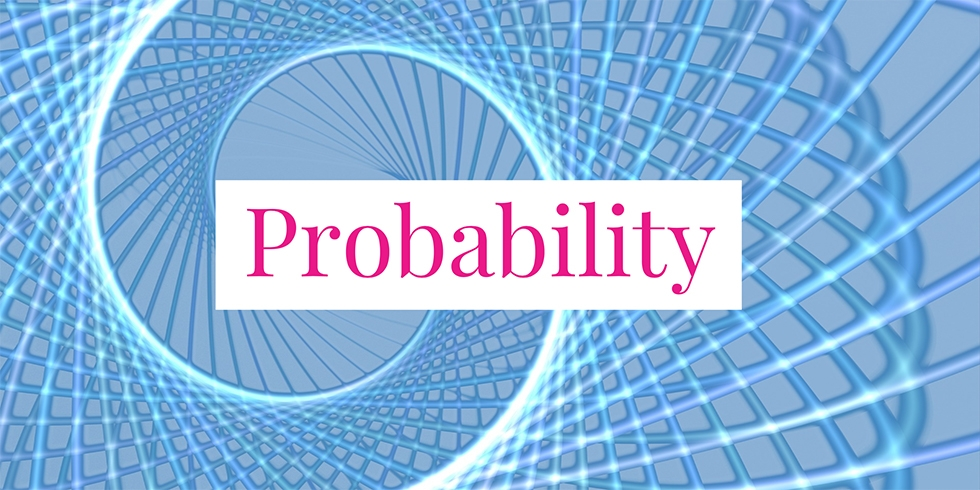# Probability Problems about Two Dice## Problem 727

Two fair and distinguishable six-sided dice are rolled.

(1) What is the probability that the sum of the upturned faces will equal $5$?

(2) What is the probability that the outcome of the second die is strictly greater than the first die?Add to solve later

## Solution.

The sample space $S$ is the set of all pairs $(i, j)$ with $1 \leq i, j \leq 6$, where $i$ and $j$ are the numbers corresponding to the first and the second die respectively. Hence $|S| = 36$. All of the $36$ possible outcomes are equally likely.

### Solution (1)

Now we consider the outcomes that give rise to the event that the sum of the upturned faces is $5$. There are four such outcomes:
$(1, 4), (2, 3), (3, 2), (4, 1).$ Thus, the desired probability is $\frac{4}{36}=\frac{1}{9}$.

### Solution (2)

The outcomes that give rise to this event are $(i, j)$ with $i < j$, where $i$ and $j$ are the numbers corresponding to the first and second die respectively. We count the number of such outcomes as follows. When $i=1$, possible values for $j$ are $j=2, 3, 4, 5, 6$. When $i=2$, possible values for $j$ are $j = 3, 4, 5, 6$. In general, $j$ can be $j = i+1, i+2, \dots, 6$. Note that $i$ cannot be $6$, otherwise $j$ is not strictly greater than $i$. Counting this way, we see that there are $5+4+3+2+1 = 15$ outcomes, each of probability $1/36$. Hence the probability that the outcomes of the second die is strictly greater than the first die is $15 \times \frac{1}{36} = \frac{15}{36} = \frac{5}{12}.$Add to solve later